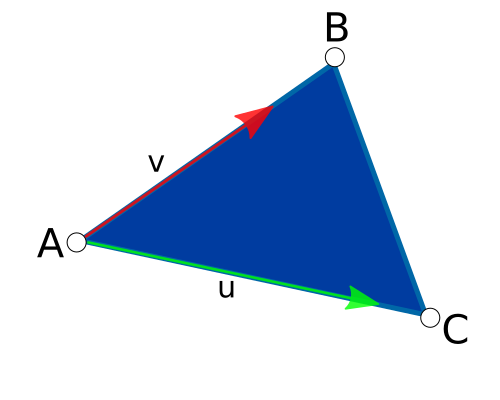## Triangle

Triangle — A triangle described by 3D points

## Functions

 graphene_triangle_t * graphene_triangle_alloc () void graphene_triangle_free () graphene_triangle_t * graphene_triangle_init_from_point3d () graphene_triangle_t * graphene_triangle_init_from_vec3 () graphene_triangle_t * graphene_triangle_init_from_float () void graphene_triangle_get_points () void graphene_triangle_get_vertices () float graphene_triangle_get_area () void graphene_triangle_get_midpoint () void graphene_triangle_get_normal () void graphene_triangle_get_plane () void graphene_triangle_get_bounding_box () bool graphene_triangle_get_barycoords () bool graphene_triangle_get_uv () bool graphene_triangle_contains_point () bool graphene_triangle_equal ()

## Includes

```#include <graphene.h>
```

## Description

graphene_triangle_t represents a triangle in 3D space.

## Functions

### graphene_triangle_alloc ()

```graphene_triangle_t *
graphene_triangle_alloc (`void`);```

Allocates a new graphene_triangle_t.

The contents of the returned structure are undefined.

[constructor]

#### Returns

the newly allocated graphene_triangle_t structure. Use `graphene_triangle_free()` to free the resources allocated by this function.

[transfer full]

Since: 1.2

### graphene_triangle_free ()

```void
graphene_triangle_free (`graphene_triangle_t *t`);```

Frees the resources allocated by `graphene_triangle_alloc()`.

Since: 1.2

### graphene_triangle_init_from_point3d ()

```graphene_triangle_t *
graphene_triangle_init_from_point3d (`graphene_triangle_t *t`,
`const graphene_point3d_t *a`,
`const graphene_point3d_t *b`,
`const graphene_point3d_t *c`);```

Initializes a graphene_triangle_t using the three given 3D points.

#### Parameters

 t the graphene_triangle_t to initialize a [nullable] b [nullable] c [nullable]

#### Returns

the initialized graphene_triangle_t.

[transfer none]

Since: 1.2

### graphene_triangle_init_from_vec3 ()

```graphene_triangle_t *
graphene_triangle_init_from_vec3 (`graphene_triangle_t *t`,
`const graphene_vec3_t *a`,
`const graphene_vec3_t *b`,
`const graphene_vec3_t *c`);```

Initializes a graphene_triangle_t using the three given vectors.

#### Parameters

 t the graphene_triangle_t to initialize a [nullable] b [nullable] c [nullable]

#### Returns

the initialized graphene_triangle_t.

[transfer none]

Since: 1.2

### graphene_triangle_init_from_float ()

```graphene_triangle_t *
graphene_triangle_init_from_float (`graphene_triangle_t *t`,
`const float *a`,
`const float *b`,
`const float *c`);```

Initializes a graphene_triangle_t using the three given arrays of floating point values, each representing the coordinates of a point in 3D space.

#### Parameters

 t the graphene_triangle_t to initialize a an array of 3 floating point values. [not nullable][array fixed-size=3] b an array of 3 floating point values. [not nullable][array fixed-size=3] c an array of 3 floating point values. [not nullable][array fixed-size=3]

#### Returns

the initialized graphene_triangle_t.

[transfer none]

Since: 1.10

### graphene_triangle_get_points ()

```void
graphene_triangle_get_points (`const graphene_triangle_t *t`,
`graphene_point3d_t *a`,
`graphene_point3d_t *b`,
`graphene_point3d_t *c`);```

Retrieves the three vertices of the given graphene_triangle_t and returns their coordinates as graphene_point3d_t.

#### Parameters

 t a return location for the coordinates of the first vertex. [out caller-allocates][optional] b return location for the coordinates of the second vertex. [out caller-allocates][optional] c return location for the coordinates of the third vertex. [out caller-allocates][optional]

Since: 1.2

### graphene_triangle_get_vertices ()

```void
graphene_triangle_get_vertices (`const graphene_triangle_t *t`,
`graphene_vec3_t *a`,
`graphene_vec3_t *b`,
`graphene_vec3_t *c`);```

Retrieves the three vertices of the given graphene_triangle_t.

#### Parameters

 t a return location for the first vertex. [out caller-allocates][optional] b return location for the second vertex. [out caller-allocates][optional] c return location for the third vertex. [out caller-allocates][optional]

Since: 1.2

### graphene_triangle_get_area ()

```float
graphene_triangle_get_area (`const graphene_triangle_t *t`);```

Computes the area of the given graphene_triangle_t.

#### Returns

the area of the triangle

Since: 1.2

### graphene_triangle_get_midpoint ()

```void
graphene_triangle_get_midpoint (`const graphene_triangle_t *t`,
`graphene_point3d_t *res`);```

Computes the coordinates of the midpoint of the given graphene_triangle_t.

The midpoint G is the centroid of the triangle, i.e. the intersection of its medians.

#### Parameters

 t res return location for the coordinates of the midpoint. [out caller-allocates]

Since: 1.2

### graphene_triangle_get_normal ()

```void
graphene_triangle_get_normal (`const graphene_triangle_t *t`,
`graphene_vec3_t *res`);```

Computes the normal vector of the given graphene_triangle_t.

#### Parameters

 t res return location for the normal vector. [out caller-allocates]

Since: 1.2

### graphene_triangle_get_plane ()

```void
graphene_triangle_get_plane (`const graphene_triangle_t *t`,
`graphene_plane_t *res`);```

Computes the plane based on the vertices of the given graphene_triangle_t.

#### Parameters

 t res return location for the plane. [out caller-allocates]

Since: 1.2

### graphene_triangle_get_bounding_box ()

```void
graphene_triangle_get_bounding_box (`const graphene_triangle_t *t`,
`graphene_box_t *res`);```

Computes the bounding box of the given graphene_triangle_t.

#### Parameters

 t res return location for the box. [out caller-allocates]

Since: 1.2

### graphene_triangle_get_barycoords ()

```bool
graphene_triangle_get_barycoords (`const graphene_triangle_t *t`,
`const graphene_point3d_t *p`,
`graphene_vec2_t *res`);```

Computes the barycentric coordinates of the given point `p` .

The point `p` must lie on the same plane as the triangle `t` ; if the point is not coplanar, the result of this function is undefined.

If we place the origin in the coordinates of the triangle's A point, the barycentric coordinates are `u`, which is on the AC vector; and `v` which is on the AB vector:The returned graphene_vec2_t contains the following values, in order:

• `res.x = u`

• `res.y = v`

#### Parameters

 t p [nullable] res return location for the vector with the barycentric coordinates. [out caller-allocates]

#### Returns

`true` if the barycentric coordinates are valid

Since: 1.2

### graphene_triangle_get_uv ()

```bool
graphene_triangle_get_uv (`const graphene_triangle_t *t`,
`const graphene_point3d_t *p`,
`const graphene_vec2_t *uv_a`,
`const graphene_vec2_t *uv_b`,
`const graphene_vec2_t *uv_c`,
`graphene_vec2_t *res`);```

Computes the UV coordinates of the given point `p` .

The point `p` must lie on the same plane as the triangle `t` ; if the point is not coplanar, the result of this function is undefined. If `p` is `NULL`, the point will be set in (0, 0, 0).

The UV coordinates will be placed in the `res` vector:

• `res.x = u`

• `res.y = v`

#### Parameters

 t p [nullable] uv_a the UV coordinates of the first point uv_b the UV coordinates of the second point uv_c the UV coordinates of the third point res a vector containing the UV coordinates of the given point `p` . [out caller-allocates]

#### Returns

`true` if the coordinates are valid

Since: 1.10

### graphene_triangle_contains_point ()

```bool
graphene_triangle_contains_point (`const graphene_triangle_t *t`,
`const graphene_point3d_t *p`);```

Checks whether the given triangle `t` contains the point `p` .

 t p

#### Returns

`true` if the point is inside the triangle

Since: 1.2

### graphene_triangle_equal ()

```bool
graphene_triangle_equal (`const graphene_triangle_t *a`,
`const graphene_triangle_t *b`);```

Checks whether the two given graphene_triangle_t are equal.

 a b

#### Returns

`true` if the triangles are equal

Since: 1.2

## Types and Values

### graphene_triangle_t

```typedef struct {
} graphene_triangle_t;
```

A triangle.

Since: 1.2